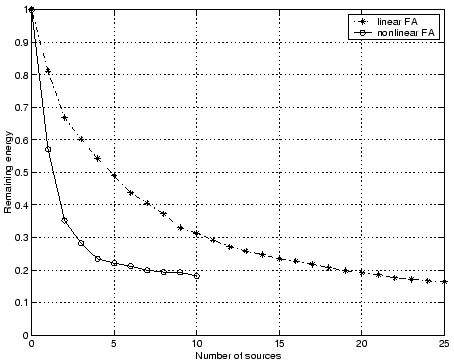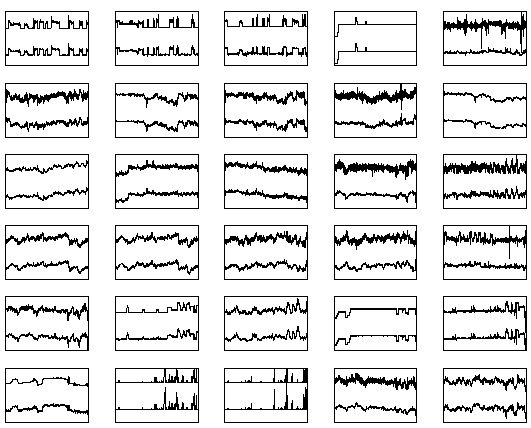Next: Comparison with Existing Methods Up: Experiments Previous: Non-Gaussian Sources.

## Process Data

This data set consists of 30 time series of length 2480 measured from different sensors from an industrial pulp process. An expert has preprocessed the signals by roughly compensating for time lags of the process which originate from the finite speed of pulp flow through the process.In order to get an idea of the dimensionality of the data, linear factor analysis was applied to the data. The result is shown in Fig. 14. The same figure shows also the results with nonlinear factor analysis. It appears that the data is quite nonlinear since the nonlinear factor analysis is able to explain as much data with 10 components as the linear factor analysis with 21 components.Several different numbers of hidden neurons and sources where tested with different random initialisations with nonlinear factor analysis and it turned out that the cost function was minimised for a network having 10 sources and 30 hidden neurons. The same network was chosen for nonlinear independent factor analysis, i.e., after 2000 iterations with linear factor analysis the sources were rotated with FastICA and each source was modelled with a mixture of three Gaussian distributions. The resulting sources are shown in Fig. 15.Figure 16 shows the 30 original time series of the data set, one time series per plot, and in the same plots below the original time series are the reconstructions made by the network, i.e., the posterior means of the output of the network when the inputs were the estimated sources shown in Fig. 15. The original signals shown great variability but the reconstructions are strikingly accurate. In some cases it even seems that the reconstruction is less noisy than the original signal. This is somewhat surprising since the time dependencies in the signal were not included in the model. The observation vectors could be arbitrarily shuffled and the model would still give the same result.

Initial studies are pointing to the direction that the estimated source signals can have meaningful physical interpretations. The results are encouraging but further studies are needed to verify the interpretations of the signals.Next: Comparison with Existing Methods Up: Experiments Previous: Non-Gaussian Sources.
Harri Lappalainen
2000-03-03